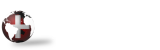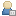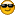####Show Posts

This section allows you to view all posts made by this member. Note that you can only see posts made in areas you currently have access to.

### Messages - GordDavey

Pages: 
1
##### Off Topic / Richter Software - Help Advice Needed
« on: August 02, 2022, 02:07:54 PM »
Hi All,

I am maintaining a 30 odd year old Richter & Associates "Sewn Package" for a customer, and I could really use some help from anyone who has a background in this package.

More specifically year end procedures / setup and change of year end from one month end to a different one. While I am fine modifying the software, I do not know much about the procedural side of the package. If one of you has a background in Richter software and can help, I will gladly pay you for some consulting time.

Gord Davey <gord.davey@daveycomputing.com>
Gord Davey Computing +1 (519) 835-4322

2
##### Nomads / Error 41 in _winproc.dsp
« on: January 22, 2021, 01:24:12 PM »
In PxPlus 17.10, I am getting an error 41 in _winproc.dsp, line 3826

Line is:
3826 if _OBJ_LISTBOX_TYPE\$="L" then let n=1; if _OBJ_EXTENSION\$<>"" then let n=num(_OBJ_EXTENSION\$,1) end_if ; if pos("RowHeight="=_OBJ_PROPS\$)=0 then if pos("M"=_OBJ_QRY_ATTR\$) then let ID'rowheight=ID'rowheight*n else let ID'LinesPerRow=n

Line should be:
3826 if _OBJ_LISTBOX_TYPE\$="L" then let n=1; if _OBJ_EXTENSION\$<>"" then let n=max(1,num(_OBJ_EXTENSION\$,1)) end_if ; if pos("RowHeight="=_OBJ_PROPS\$)=0 then if pos("M"=_OBJ_QRY_ATTR\$) then let ID'rowheight=ID'rowheight*n else let ID'LinesPerRow=n

An older panel the _OBJ_EXTENSION\$ was = "0" and it would cause n=0 in this line, which would lead to an error message when the panel was first drawn. Wrapping it with a MAX(1,num(_OBJ_EXTENSION\$,1)) resolves the issue.

Gord.

3
##### Programming / Re: Binary auto increment key
« on: October 05, 2020, 05:55:45 PM »
Michael,

The var which holds the auto increment must be blank, when you write the record. You can then read the record with a KEC() to get it back and the var should have the new value in it.

4
##### Nomads / Re: delete items from a listbox with <DEL>
« on: March 19, 2020, 10:06:25 AM »
Thomas,

If you are using Nomads, then take a look at the _EOM\$ var. It will have, \$2E\$ for the delete key... (I think it was \$2E\$ I might be off a bit).
If not using nomads, then there is a string var in the syntax of the LIST_BOX READ which will return the eom value.

5
##### Thin Client/WindX / Re: Simple client over remote rdp not connecting sometimes
« on: March 18, 2020, 08:30:46 AM »
Does the server that is seeing a slow start of the pvxplus.exe have a connection to the internet? If it does then does it limit outbound connections in any way? Sites/Sockets ?

6
##### Programming / Re: GUID generator
« on: December 05, 2019, 04:59:15 PM »
Dave,
If you are using Windows then the following OOP method will return new GUID's in hex and in lowercase, which is typically how they are stored, since you don't want to store the 16 binary chars that make them up as you could have field seps in the binaary values.:

function local getGUID(guid\$)
local pGuid\$, S_OK, BYTE
SO_OK = 0, BYTE = tcb(303)
enter pGuid\$

pGuid\$ = dim(BYTE * 16, \$00\$)
if dll("Ole32", "CoCreateGuid", pGuid\$) <> S_OK pGuid\$ = ""; return 0
pGuid\$ = lcs(hta(pGuid\$))

return 1

7
##### Language / Re: conversion of ADJN(
« on: March 28, 2019, 11:05:10 AM »
Lawrence, BBx's docs say it returns an 8 byte binary string. Perhaps you can test: PRINT HTA(BIN(BIGNUMBER,) and see if BIN gives you the same value when you use HTA to convert it to hex so you can see it ?

8
##### Programming / Re: ZPL II Programming in PxPlus
« on: December 18, 2018, 05:13:27 PM »

Data\$ = ""
Data\$ += "^XA^LRN^CI0^XZ" ! start format, reverse image, FontEncoding, stop format
Data\$ += "^XA" ! Start format
Data\$ += "^MMT^JZY" ! Tearoff, reprint on error
Data\$ += "^LT0^PON^PMN^LH0,0^JMA" !
Data\$ += "^PW812" ! Label Width in dots (from 203 dot GK420T)
Data\$ += "^LL1218" ! Label Length in dots (from 203 dot GK420T)
Data\$ += "^LS0" ! Label shift to 0
Data\$ += "^FO34,750^GB738,417,8^FS" ! Draw Box
Data\$ += "^FT619,985^A0I,169,196^FH\^FDSTOP^FS" ! ! STOP
Data\$ += "^FT724,820^A0I,118,122^FH\^FDDo Not Apply^FS" ! DO NOT APPY
Data\$ += "^FT507,665^A0I,66,70^FH\^FD" + This'FormatContainerID\$(ContainerID\$, 0) + "^FS" ! Human Readable Bar Code Value - ContainerID last 6 digits

if CompanyCode\$ > "" \
then Data\$ += "^FT770,560^A0I,51,52^FH\^FD" + "Inv: " + CompanyCode\$ + " " + InvoiceNo\$ + " " + This'DisplayDate\$(InvoiceDate\$) + "^FS" ! Text Comp InvoiceNo InvoiceDate

if CustomerCode\$ > "" then \
{
tmp\$ = stp(stp(CustomerAbbrev\$, 2) + " " + CustomerCode\$ + "-" + ShipToCode\$ + " " + ShipToProvAbbrev\$, 2)
Data\$ += "^FT770,495^A0I,51,52^FH\^FD" + "Cst: " + tmp\$ + "^FS" ! Text CustAbbv, Cust#, ShipTo#, Prov
}

Data\$ += "^FT770,395^A0I,51,52^FH\^FDError:^FS" ! Text Error
Data\$ += "^FT630,395^A0I,51,52^FH\^FD" + str(ErrorCode) + "^FS" ! Text ErrorCode

! Error Text, we need to watch out for how LONG the text is
! Seems to be 40 chars per line, max 5 lines
tmp\$ = stp(ErrorText\$, 2), line = 0
dim line\$[ 1 : 5 ]

while tmp\$ > ""
if line >= 5 \
then break

if len(tmp\$) <= 33 then \
{
Line\$[ ++line ] = tmp\$, \
tmp\$ = ""
} else \
{
x = pos(" ,." : tmp\$(1, 34), -1)
if x >= 18 then \
{
Line\$[ ++line ] = stp(mid(tmp\$, 1, x - 1))
tmp\$ = stp(mid(tmp\$, x + 1), 2)
} else \
{
Line\$[ ++line ] = stp(mid(tmp\$, 1, 33))
tmp\$ = stp(mid(tmp\$, 34), 2)
}
}
wend

LineY = 330
if Line\$ > "" \
then Data\$ += "^FT770," + str(LineY) + "^A0I,51,52^FH\^FD" + Line\$ + "^FS"; LineY -= 65 ! Text ErrorText Line 1
if Line\$ > "" \
then Data\$ += "^FT770," + str(LineY) + "^A0I,51,52^FH\^FD" + Line\$ + "^FS"; LineY -= 65 ! Text ErrorText Line 1
if Line\$ > "" \
then Data\$ += "^FT770," + str(LineY) + "^A0I,51,52^FH\^FD" + Line\$ + "^FS"; LineY -= 65 ! Text ErrorText Line 1

Data\$ += "^PQ1,0,1,Y^XZ" ! Print Quality 1, pause&cut, replicates, Override pause count

Pages: